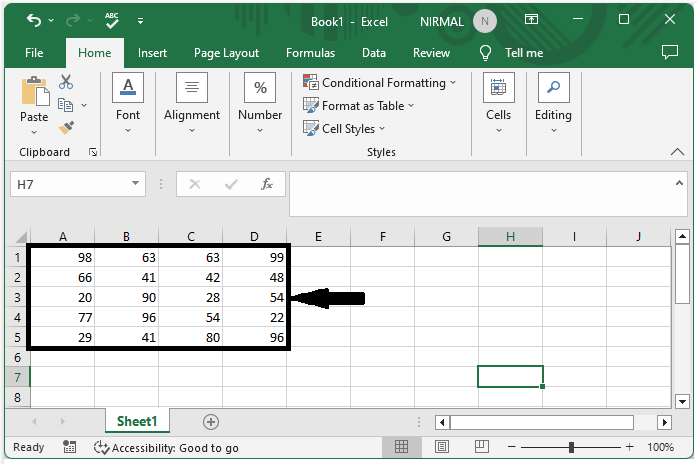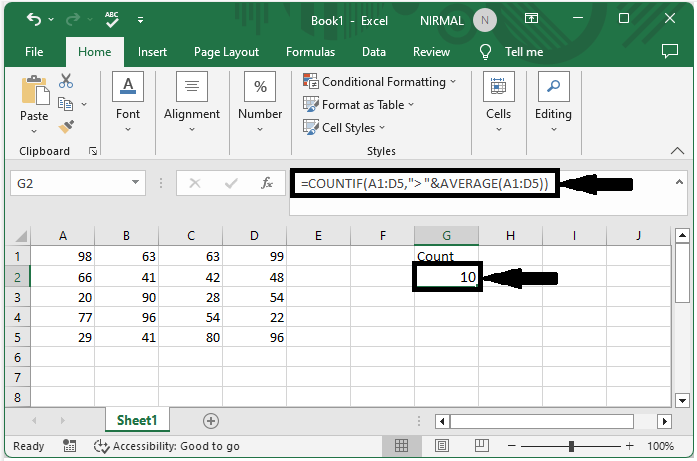# How To Count The Cells Above Certain Value Or Average In Excel

Microsoft Excel is a powerful tool that has revolutionized the way we process and analyze data. One common task that you may encounter when working with Excel is counting the number of cells that meet a certain criterion, such as being above a certain value or above the average of a range of cells.

Counting cells in Excel can be done using various built-in functions, such as COUNTIF and COUNTIFS, which allow you to count cells based on a specific condition. In this tutorial, we will focus on using these functions to count cells that meet specific criteria. Whether you are a student, researcher, or professional, this tutorial will provide you with a step-by-step guide on how to count cells above a certain value or average in Excel, enabling you to better understand and analyze your data. So, let's get started!

## Count The Cells Above Certain Value Or Average

Here we will first get the count directly using a single formula. So let us see a simple process to know how you can count the cells above a certain value or average in Excel.

### Step 1

Consider an Excel sheet where you have a collection on a number, similar to the below image.First, click on an empty cell and enter the formula as =COUNTIF(A1:D5,"> "&AVERAGE(A1:D5)) and click enter to get the count. In the formula, A1:D5 is the range of cells containing numbers.

Empty cell > Formula > Enter.Note

If you want to count cells below the average, we can use the formula =COUNTIF(A1:D5,"< "&AVERAGE(A1:D5)) and click enter, or use the formula =COUNTIF(A1:C5,"<30 ") to count the cells above a specified value.

## Conclusion

In this tutorial, we used a simple example to demonstrate how you can count the cells above a certain value or average in Excel to highlight a particular set of data.

Updated on: 12-Jul-2023

187 Views